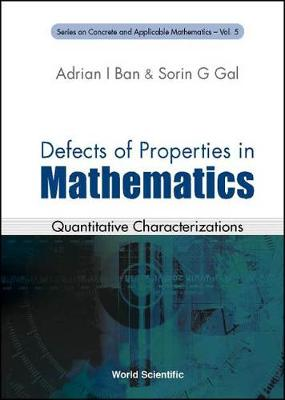•Defects Of Properties In Mathematics: Quantitative Characterizations - Series on Concrete & Applicable Mathematics 5 (Hardback)

(author), (author)
£112.00
Hardback 364 Pages / Published: 23/04/2002
• We can order this

Usually dispatched within 3 weeks

This book introduces a method of research which can be used in various fields of mathematics. It examines, in a systematic way, the quantitative characterizations of the "deviation from a (given) property", called the "defect of a property", in: set theory; topology; measure theory; real, complex and functional analysis; algebra; geometry; number theory; fuzzy mathematics.Besides well-known "defects", the book introduces and studies new ones, such as: measures of noncompactness for fuzzy sets; fuzzy and intuitionistic entropies; the defect of (sub, super)additivity; complementarity; monotonicity for set functions; the defect of convexity; monotonicity; differentiability for real functions; the defect of equality for inequalities; the defect of orthogonality for sets and defects of properties for linear operators in normed spaces; defects of properties (commutativity, associativity, etc.) for binary operations; defects of orthogonality and parallelness in Euclidean and non-Euclidean geometries; defects of integer, perfect, prime and amicable numbers; the defect of tautology in fuzzy logic.

Publisher: World Scientific Publishing Co Pte Ltd
ISBN: 9789810249243
Number of pages: 364